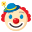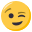# std::vector of structs using operator ==

• Hey,

I got a std::vector of self-defined structs.
I have implemented the ==operator for my struct. I thought comparing two vectors vec1==vec2 would work like that, but it doesn't.

can anybody tell me if I'm doing something wrong or do I just have to iterate through the vector and compare each of the elements singularily?

here's my code.
thanks a lot.

``````#include<iostream>
#include<vector>
using namespace std;

struct A
{
int a;
bool operator==(const A &other){
if(a==other.a)
return true;
return false;
}
};

int main()
{
vector<A> a;
vector<A> b;

A a1;
A a2;
a1.a=1;
a2.a=1;
a.push_back(a1);
b.push_back(a2);
if(a==b)
cout<<"a,b equal"<<endl;
else
cout<<"a,b not equal"<<endl;

return 0;
}
``````

• The operator == is not overloaded for std::vector. You could do that yourself

``````template <typename T>
bool operator == (const std::vector<T>& lhs, const std::vector<T>& rhs)
{
return lhs.size() == rhs.size() && std::equal(lhs.begin(), lhs.end(), rhs.begin());
}
``````

or just write the code out as shown above.

• One thing you have to keep in mind is that overloading operator== for std::vector in the std namespace is illegal and if you do it somewhere else, you won't get the benefit of ADL (argument dependent lookup). So, maybe, a name different from operator== for a function like this is appropriate since people tend to expect such operators to "just work" without worrying about name lookup.

• Annah.Do schrieb:

``````struct A
{
int a;
bool operator==(const A &other){
if(a==other.a)
return true;
return false;
}
};
``````

You forgot the 'const' behind the operator==()-method
better:

``````struct A
{
int a;
bool operator==(const A &other) const { // const-methode
if(a==other.a)
return true;
return false;
}
};
``````

cooky451 schrieb:

The operator == is not overloaded for std::vector.

C++-Standard - chapter '23.2.1 General container requirements' - Table 96 'Container requirements': a == b
In Tables 96 and 97, X denotes a container class containing objects of type T, a and b denote values of type X

:xmas2:

• By the way, this is a valid quote for C++98 and C++11 (only the Chapter is a little bit different in 98, it's 32.1 there) :xmas1:

• cooky451 schrieb:

The operator == is not overloaded for std::vector. You could do that yourself

``````template <typename T>
bool operator == (const std::vector<T>& lhs, const std::vector<T>& rhs)
{
return lhs.size() == rhs.size() && std::equal(lhs.begin(), lhs.end(), rhs.begin());
}
``````

Since i am pretty meticulous, i wouldn't consider this the best solution, since your's doesn't allow other Allocators than the default one (which is the standard allocator).``````template <typename T,
typename A>
bool operator == (std::vector<T, A> const& lhs,
std::vector<T, A> const& rhs)
{
return lhs.size() == rhs.size()
&& std::equal(lhs.begin(), lhs.end(), rhs.begin());
}

template <typename T,
typename A>
bool operator!=(std::vector<T, A> const& lhs,
std::vector<T, A> const& rhs)
{
return !(lhs == rhs);
}
``````

usually
Additionally, as you can see, i added the inequality operator. Just because this usually always comes with it's partner, the equality op. :xmas2:

Edit:

Werner_logoff schrieb:

Annah.Do schrieb:

``````struct A
{
int a;
bool operator==(const A &other){
if(a==other.a)
return true;
return false;
}
};
``````

You forgot the 'const' behind the operator==()-method
better:

``````struct A
{
int a;
bool operator==(const A &other) const { // const-methode
if(a==other.a)
return true;
return false;
}
};
``````

IMO even better:

``````struct A
{
int a;
};

bool operator==(A const& a,
A const& b)
{
return a.a == b.a;
}
``````

:xmas1:
Edit²: Well, in this particular case, a member definition would be totally fine. I just thought, you know, maybe there is an implicit conversion ctor in the "real" version...

• Werner_logoff schrieb:

cooky451 schrieb:

The operator == is not overloaded for std::vector.

C++-Standard - chapter '23.2.1 General container requirements' - Table 96 'Container requirements': a == b
In Tables 96 and 97, X denotes a container class containing objects of type T, a and b denote values of type X
:xmas2:

Should've looked it up